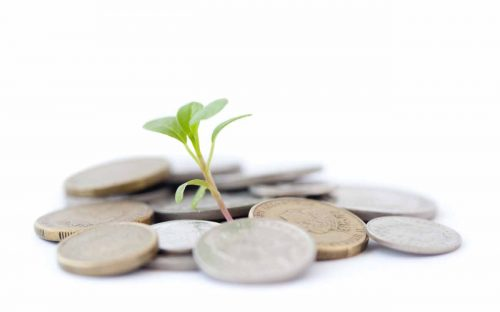# Rental Yield CalculatorWhat Is Gross Yield?

Gross Yield is the annual return on investment before the deduction of taxes and expenses. Gross yield is usually expressed in percentage terms

How to Calculate Gross Yield?

Step 1. Take the rental price of a property per calendar month. Step 2. Multiple this by 12 to obtain the annual rent Step 3. Take the annual rent and divide it by the actual or proposed purchase price of the property. Step 4. Multiply this figure by 100, to move the decimal point to the correct position. This will now be a percentage.

Examples –

Rent p/m £750 x 12/£150,000 = Gross yield 6.00%

Rent p/m £850 x 12/£200,000 = Gross yield 5.10% Net Yield is the annual return on investment after the deduction of taxes and expenses.

# Rental Yield Calculator

(Enter numbers only)